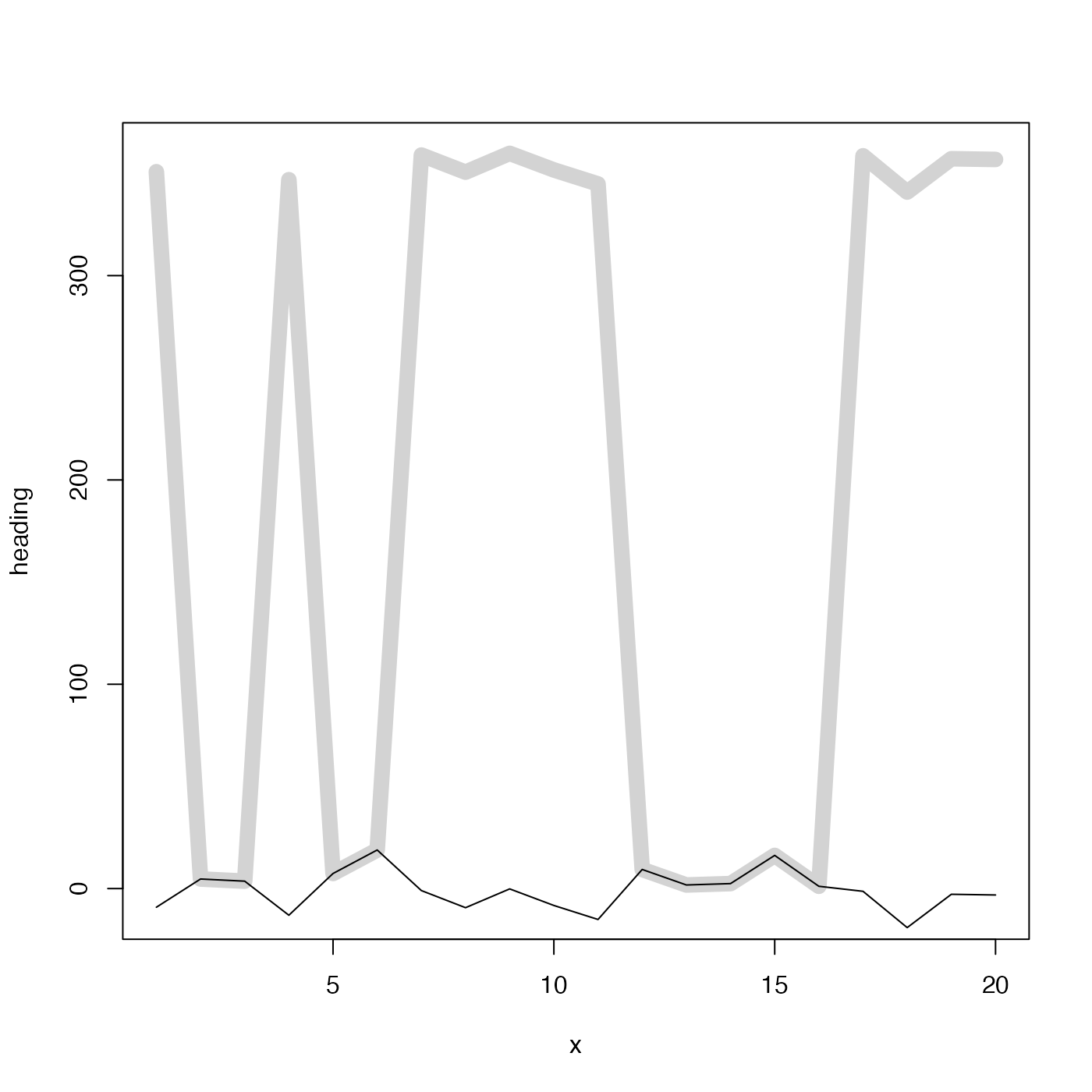This is mostly used for instrument heading angles, in cases where the instrument is aligned nearly northward, so that small variations in heading (e.g. due to mooring motion) can yield values that swing from small angles to large angles, because of the modulo-360 cut point. The method is to use the cosine and sine of the angle in order to find "x" and "y" values on a unit circle, and then to use atan2() to infer the angles.

angleRemap(theta)

## Arguments

theta an angle (in degrees) that is in the range from 0 to 360 degrees

## Value

A vector of angles, in the range -180 to 180.

## Examples


library(oce)
## fake some heading data that lie near due-north (0 degrees)
n <- 20
heading <- 360 + rnorm(n, sd=10)
plot(x, heading, ylim=c(-10, 360), type='l', col='lightgray', lwd=10)lines(x, angleRemap(heading))